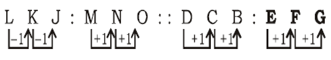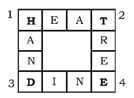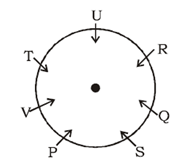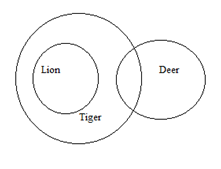# SSC CGL 2018 Practice Test Papers | Reasoning Ability (Day-41)

Dear Aspirants, Here we have given the Important SSC CGL Exam 2018 Practice Test Papers. Candidates those who are preparing for SSC CGL 2018 can practice these questions to get more confidence to Crack SSC CGL 2018 Examination.

[WpProQuiz 3293]

Click “Start Quiz” to attend these Questions and view Explanation

1) Select set of letters from the given alternatives which when sequentially placed at the gaps in the given letter series, complete it. xx_zzyx_yzzyxxy_zy_xyzz_

a) yxzxy

b) yxzyx

c) yxxyz

d) xxzyy

2) If ‘−’ stands for ‘×’, ‘×’ stands for ‘+’, ‘+’ stands for ‘÷’, and ‘÷’ stands for ‘−’, then

42 + 7 – 6 ÷ 5 × 14 =?

a) 36

b) 40

c) 43

d) 45

3) Safe: Secure :: Armour: ?

a) Calm

b) Danger

c) Weapon

d) Rule

4) Select the related word/letters/number from the given alternatives.

LKJ : MNO : : DCB : ?

a) FEG

b) EGF

c) FGH

d) EFG

5) Find the odd/ word/letters/ number from the given alternatives.

729, 784, 841, 900, 1094

a) 841

b) 729

c) 784

d) 1094

6) Choose the correct alternative from the given ones that will complete the series.

2, 5, 10, 50, ?, 2500

a) 60

b) 600

c) 50

d) 500

7) In the given puzzle, which of the letters from the given option will form meaningful words if placed on the corners 1, 2, 3 and 4?

a) LNRG

b) FLPK

c) HTDE

d) HPGT

(8-9) P, Q, R, S, T, U and V are sitting around a circle facing the centre. P is exactly between V and S. R, who is second to the right of S, is between Q and U. Q is not the neighbour of T.

8) Which of the following statements is false?

a) R is to the immediate left of U

b) Q is to the immediate left to R

c) T is third to the right of Q

d) U is second to the left of T

9) What is the position of Q with respect to V?

a) Third to the right

b) Second to the left

c) Immediate left

d) Third to the left

10) Statement:

All lions are tiger.

Some tiger are deer.

Conclusion:

1. No deer is tiger
2. Some deer is tiger

a) Only conclusion I follow.

b) Only conclusion II follows.

c) Both I and II follows.

d) Either I or II follows

The given series is formed by repeating x x y z z y / x x y z z y / x x y z z y / x x y z z y

42 + 7 – 6 ÷ 5 × 14

= 42 ÷ 7 × 6 – 5 + 14

= 42 ÷ 7 × 6 – 5 + 14

= 6 × 6 – 5 + 14

= 36 – 5 + 14

= 45

Safe and Secure are synonyms. Armour and Weapon are synonyms.729 = 272           784 = 282         841 = 282         900 = 302

1096 is not a square number

2          5×2       10×5     50×10   500×50            2500

Multiply by previous numbers